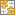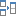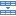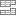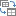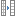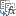# How to use VLOOKUP with SUM or SUMIF functions in Excel

In this tutorial, you will find a handful of advanced formula examples that demonstrate how to use Excel's VLOOKUP and SUM or SUMIF functions to look up and sum values based on one or several criteria.

Are you trying to create a summary file in Excel that will identify all instances of one particular value, and then sum other values that are associated with those instances? Or, do you need to find all values in an array that meet the condition you specify and then sum the related values from another worksheet? Or maybe you are faced with a more concrete challenge, like looking through a table of your company invoices, identifying all invoices of a particular vendor, and then summing all the invoice values?

The tasks may vary, but the essence is the same - you want to look up and sum values with one or several criteria in Excel. What kind of values? Any numeric values. What sort of criteria? Any : ) Starting from a number or reference to a cell containing the right value, and ending with logical operators and results returned by Excel formulas.

So, does Microsoft Excel have any functionality that can help with the above tasks? Of course, it does! You can work out a solution by combining Excel's VLOOKUP or LOOKUP with SUM or SUMIF functions. The formula examples that follow below will help you understand how these Excel functions work and how to apply them to real data.

Please note, these are advanced examples that imply you are familiar with the general principles and syntax of the VLOOKUP function. If not, the first part of our VLOOKUP tutorial for beginners is certainly worth your attention - Excel VLOOKUP syntax and general usages.

## Excel VLOOKUP and SUM - find the sum of matching values

If you work with numerical data in Excel, quite often you have not just to extract associated values from another table but also sum numbers in several columns or rows. To do this, you can use a combination of the SUM and VLOOKUP functions as demonstrated below.

Source data:

Suppose, you have a product list with sales figures for several months, a column per each month. The source data is on the sheet named Monthly Sales: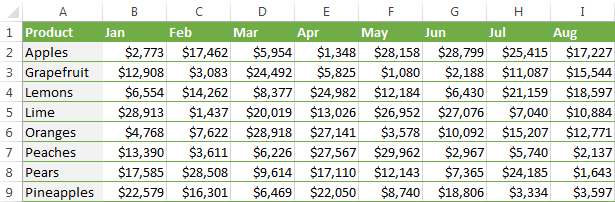Now, you want to make a summary table with the total sales for each product.

The solution is to use an array in the 3rd parameter (col_index_num) of the Excel VLOOKUP function. Here is a generic formula:

SUM(VLOOKUP(lookup value, lookup range, {2,3,...,n}, FALSE))

As you see, we use an array constant in the third argument to perform several lookups within the same VLOOKUP formula in order to get the sum of values in columns 2,3 and 4.

And now, let's adjust this combination of VLOOKUP and SUM functions for our data to find the total of sales in columns B - M in the above table:

`=SUM(VLOOKUP(B2, 'Monthly sales'! \$A\$2:\$M\$9, {2,3,4,5,6,7,8,9,10,11,12,13}, FALSE))`

Important! Since you are building an array formula, be sure to hit Ctrl + Shift + Enter instead of a simple Enter keystroke when you finished typing. When you do this, Microsoft Excel encloses your formula in curly braces like this:

`{=SUM(VLOOKUP(B2, 'Monthly sales'!\$A\$2:\$M\$9, {2,3,4,5,6,7,8,9,10,11,12,13}, FALSE))}`

If you press the Enter key as usual, only the first value in the array will get processed, which will produce incorrect results.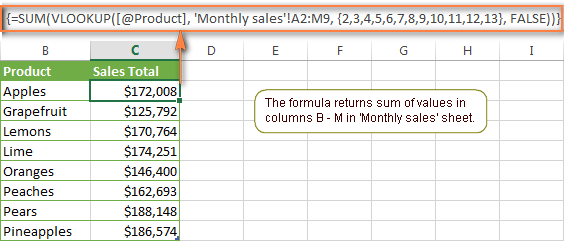Tip. You may be curious why the formula displays [@Product] as the lookup value in the screenshot above. This is because I converted my data to table (Insert tab > Table). I find it very convenient to work with fully-functional Excel tables and their structured references. For example, when you type a formula into one cell, Excel automatically copies it across the entire column and in this way saves you a few precious seconds :)

As you see, using the VLOOKUP and SUM functions in Excel is easy. However, this is not the ideal solution, especially if you are working with big tables. The point is that using array formulas may adversely affect the workbook's performance since each value in the array makes a separate call of the VLOOKUP function. So, the more values you have in the array and the more array formulas you have in your workbook, the slower Excel works.

You can bypass this problem by using a combination of the INDEX and MATCH functions instead of SUM and VLOOKUP, and I will show you a few formula examples in the next article.

## How to perform other calculations with Excel VLOOKUP function

A moment ago we discussed an example of how you can extract values from several columns in the lookup table and calculate the sum of those values. In the same fashion, you can perform other mathematical calculations with the results returned by the VLOOKUP function. Here are a few formula examples:

 Operation Formula example Description Calculate average {=AVERAGE(VLOOKUP(A2, 'Lookup Table'\$A\$2:\$D\$10, {2,3,4}, FALSE))} The formula searches for the value of cell A2 in 'Lookup table' and calculates the average of values in columns B,C and D in the same row. Find maximum value {=MAX(VLOOKUP(A2, 'Lookup Table'\$A\$2:\$D\$10, {2,3,4}, FALSE))} The formula searches for the value of cell A2 in 'Lookup table' and finds the max value in columns B,C and D in the same row. Find minimum value {=MIN(VLOOKUP(A2, 'Lookup Table'\$A\$2:\$D\$10, {2,3,4}, FALSE))} The formula searches for the value of cell A2 in 'Lookup table' and finds the min value in columns B,C and D in the same row. Calculate % of sum {=0.3*SUM(VLOOKUP(A2, 'Lookup Table'\$A\$2:\$D\$10, {2,3,4}, FALSE))} The formula searches for the value of cell A2 in 'Lookup table', sums values in columns B,C and D in the same row, and then calculates 30% of the sum.
Note. Since all of the above formulas are array formulas, remember to press Ctrl+Shift+Enter to enter them correctly in a cell.

If we add the above formulas to the 'Summary Sales' table from the previous example, the result will look similar to this: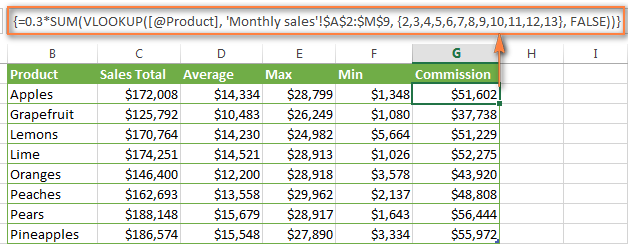## LOOKUP AND SUM - look up in array and sum matching values

In case your lookup parameter is an array rather than a single value, the VLOOKUP function is of no avail because it cannot look up in data arrays. In this case, you can use Excel's LOOKUP function that is analogues to VLOOKUP but works with arrays as well as with individual values.

Let's consider the following example, so that you can better understand what I'm talking about. Suppose, you have a table that lists customer names, purchased products and quantity (Main table). You also have a second table containing the product prices (Lookup table). Your task is to make a formula that finds the total of all orders made by a given customer.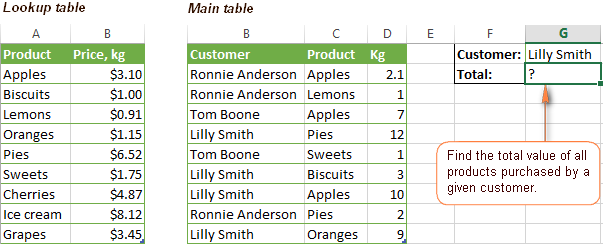As you remember, you cannot utilize the Excel VLOOKUP function since you have multiple instances of the lookup value (array of data). Instead, you use a combination of SUM and LOOKUP functions like this:

`=SUM(LOOKUP(\$C\$2:\$C\$10,'Lookup table'!\$A\$2:\$A\$16,'Lookup table'!\$B\$2:\$B\$16)*\$D\$2:\$D\$10*(\$B\$2:\$B\$10=\$G\$1))`

Since this is an array formula, remember to press Ctrl + Shift + Enter to complete it.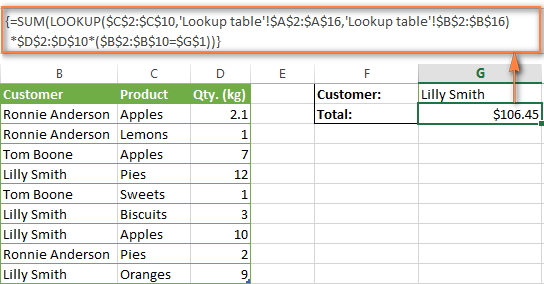And now, let's analyses the formula's ingredients so that you understand how each of the functions works and can to tweak it for your own data.

We'll put aside the SUM function for a while, because its purpose is obvious, and focus on the 3 components that are multiplied:

1. `LOOKUP(\$C\$2:\$C\$10,'Lookup table'!\$A\$2:\$A\$16,'Lookup table'!\$B\$2:\$B\$16)`

This LOOKUP function looks up the goods listed in column C in the main table, and returns the corresponding price from column B in the lookup table.

2. `\$D\$2:\$D\$10`

This component returns quantity of each product purchased by each customer, which is listed in column D in the main table. Multiplied by the price, which is returned by the LOOKUP function above, it gives you the cost of each purchased product.

3. `\$B\$2:\$B\$10=\$G\$1`

This formula compares the customers' names in column B with the name in cell G1. If a match is found, it returns "1", otherwise "0". You use it simply to "cut off" customers' names other than the name in cell G1, since all of us know that any number multiplied by zero is zero.

Because our formula is an array formula it iterates the process described above for each value in the lookup array. And finally, the SUM function sums the products of all multiplications. Nothing difficult at all, it is?

Note. For the LOOKUP formula to work correctly you need to sort the lookup column in your Lookup table in ascending order (from A to Z). If sorting is not acceptable on your data, check out an awesome SUM / TRANSPOSE formula suggested by Leo.

## VLOOKUP and SUMIF - look up & sum values with criteria

Excel's SUMIF function is similar to SUM we've just discussed in the way that it also sums values. The difference is that the SUMIF function sums only those values that meet the criteria you specify. For example, the simplest SUMIF formula `=SUMIF(A2:A10,">10")` adds the values in cells A2 to A10 that are larger than 10.

This is very easy, right? And now let's consider a bit more complex scenario. Suppose you have a table that lists the sales persons' names and ID numbers (Lookup_table). You have another table that contains the same IDs and associated sales figures (Main_table). Your task is to find the total of sales made by a given person by their ID. At that, there are 2 complicating factors:

• The mail table contains multiple entries for the same ID in a random order.
• You cannot add the "Sales person names" column to the main table.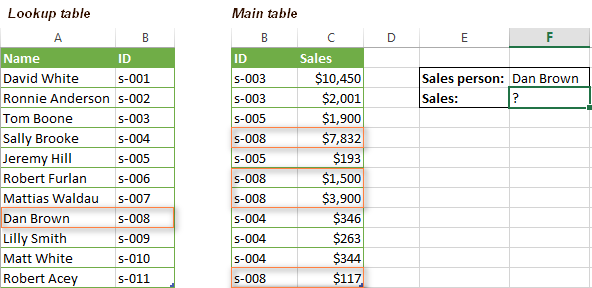And now, let's make a formula that, firstly, finds all sales made by a given person, and secondly, sums the found values.

Before we start on the formula, let me remind you the syntax of the SUMIF function:

SUMIF(range, criteria, [sum_range])
• `range` - this parameter is self-explanatory, simply a range of cells that you want to evaluate by the specified criteria.
• `criteria` - the condition that tells the formula what values to sum. It can be supplied in the form of a number, cell reference, expression, or another Excel function.
• `sum_range` - this parameter is optional, but very important to us. It defines the range where the corresponding cells' values shall be added. If omitted, Excel sums the values of cells that are specified in the range argument (1st parameter).

Keeping the above info in mind, let's define the 3 parameters for our SUMIF function. As you remember, we want to sum all the sales made by a given person whose name is entered in cell F2 in the main table (please see the image above).

1. Range - since we are searching by sales person ID, the range parameter for our SUMIF function is column B in the main table. So, you can enter the range B:B, or if you convert you data to a table, you can use the column's name instead: `Main_table[ID]`
2. Criteria - because we have sales persons' names in another table (lookup table), we have to use the VLOOKUP formula to find the ID corresponding to a given person. The person's name is written in cell F2 in the main table, so we look it up using this formula: `VLOOKUP(\$F\$2,Lookup_table,2,FALSE)`

Of course, you could enter the name in the lookup criteria of your VLOOKUP function, but using an absolute cell reference is a better approach because this creates a universal formula that works for any name input in a given cell.

3. Sum range - this is the easiest part. Since our sales numbers are in column C named "Sales", we simply put `Main_table[Sales]`.

Now, all you need is to assemble the formula's parts and your SUMIF + VLOOKUP formula is ready:

`=SUMIF(Main_table[ID], VLOOKUP(\$F\$2, Lookup_table, 2, FALSE), Main_table[Sales])`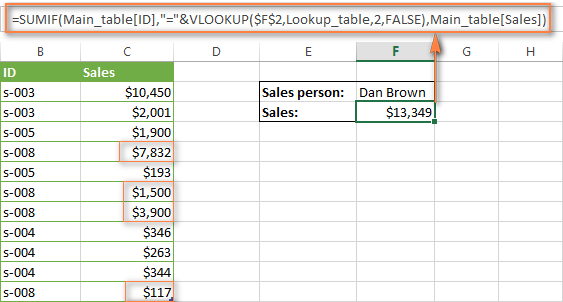## Formula-free way to do vlookup in Excel

Finally, let me introduce you to the tool that can look up, match and merge your tables without any functions or formulas. The Merge Tables tool included with our Ultimate Suite for Excel was designed and develop as a time-saving and easy-to-use alternative to Excel's VLOOKUP and LOOKUP functions, and it can be very helpful both to beginners and advanced users.

Instead of figuring out formulas, you simply specify your main and lookup tables, define a common column or columns, and tell the wizard what data you want to fetch.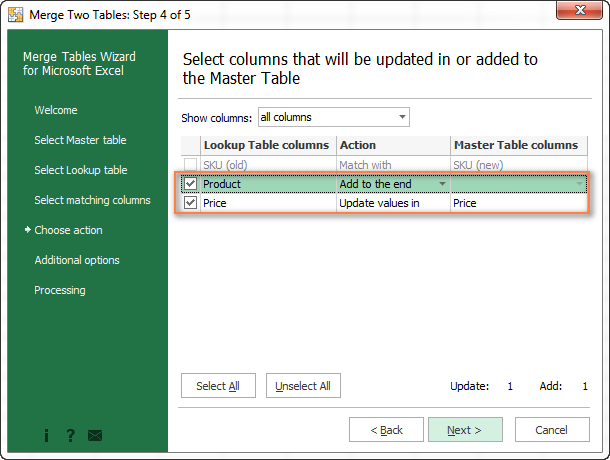Then you allow the wizard a few seconds to look up, match and deliver you the results. If you think this add-in may prove helpful in your work, you are most welcome to download a trial version by using the below link.

VLOOKUP with SUM and SUMIF - formula examples (.xlsx file)
Ultimate Suite - trial version (.zip file)

### 340 responses to "How to use VLOOKUP with SUM or SUMIF functions in Excel"

1.Jay says:

Want to do vlookup with checking lookup values and if dupilcates values find then it balance the duplicates values,else it write as single values.

E.g. A B Qty output
a1 d123 1500 1000
a2 d123 700 1000
a3 d123 400 600
a4 d124 200 200
a5 d125 300 300

•Mihna says:

A) Complete the cost, subtotal and total of the follwing table using the corresponding formulas.

"B) Introduce today's date using the corresponding formula next to the cell ""Cost"" "

C) Change the currency from euros to Pound Sterling

Quantity Description Unit Price Cost
3 Valve 1,500 €
5 Column 500,000 €
6 Clamp 980 €
2 Turbine 200,000 €
1 Compressor 100,000 €
2 Tank 300,500 €
3 Tube 6,750 €

Subtotal
VAT 21%
TOTAL

2.imran says:

I have many columns like; date, ID, fullname, customer_type, any_details

Now I want to make a form to add/ edit/ search/ delete data, Some time I would have to sum up customer type in a specific dates or month or year.

3.Paul says:

Column Column Column
A B C
Karim 100 200
Rohim 200 300
Karim 100 500
Rohim 500 100

Result should be:

Karim 900 (100+200+100+500)
Rohim 1100

I used = sumproduct(Vlookup (A1,A1:C4,{2,3},0))
But it does not work. How can I do it? Pls help

•Krishna Tamrakar says:

Yes! Paul, Same Problem With Me :), And I Need Distinct Name in Drop-down List
A B C
1 2019.12.02 Ronaldo 50
2 2019.12.02 Messi 20
3 2019.12.03 Ronaldo 50
4 2019.12.03 Messi 22
5 2019.12.04 Pele 15
6 2019.12.04 Ronaldo 44

•Ken says:

use data validation function

4.Zahirul says:

5.Juhil Lapsiwala says:

Sir,
I have applied this in rec.sheet and value voolup sum from 3 sheets.
=SUMIF(Rec.Sheet!\$B\$4:\$B\$144,B4,'Q-1'!\$J\$4:\$J\$142)+SUMIF(Rec.Sheet!\$B\$4:\$B\$144,B4,'Q-2'!\$J\$4:\$J\$142)+SUMIF(Rec.Sheet!\$B\$4:\$B\$144,B4,'Q-2'!\$J\$4:\$J\$142)+SUMIF(Rec.Sheet!\$B\$4:\$B\$144,B4,'Q-3'!\$J\$4:\$J\$142)
This works fine but need better way.
Plz give me better suggestion

6.Ana Domingo says:

Hi,

I have the chart below

Employee name:
Sick Taken :
Vacation Taken

Staff Name Type # of days From To Notes
Josephine Domingo Vacation 1.0 1-Jan-18 1-Jan-18 Approved
May Flower Vacation 0.5 1-Jan-18 1-Jan-18 Approved
Josephine Domingo Sick 1.0 1-Jan-18 1-Jan-18 Approved
May Flower Vacation 3.0 1-Jan-18 1-Jan-18 Approved
Josephine Domingo Vacation 4.0 1-Jan-18 1-Jan-18 Approved

What formula can I use to calculate the sum of Vacation days or Sicks days for a specific employee?

Any help will be helpful :)

7.Asher says:

I need to get the values of (A1 Staff costs) in by month in below table. please help me

12/20/18 A1 Staff costs 4,412.00
12/20/18 A1 Staff costs 30,232.00
12/20/18 A1 Staff costs 4,412.00
12/20/18 A1 Staff costs 141,000.00
01/10/19 B6 Travel 124.25
01/10/19 B6 Travel 3,890.19
01/10/19 B6 Travel 570.00
01/10/19 B6 Travel 401.00
01/10/19 B6 Travel 3,381.50
01/10/19 A1 Staff costs 6,300.00
01/10/19 A1 Staff costs 7,613.66

8.Asher says:

I need to get the values of (A1 Staff costs) in by month in below table. please help me

12/20/18 A1 Staff costs 4,412.00
12/20/18 A1 Staff costs 30,232.00
12/20/18 A1 Staff costs 4,412.00
12/20/18 A1 Staff costs 141,000.00
01/10/19 B6 Travel 124.25
01/10/19 B6 Travel 3,890.19
01/10/19 B6 Travel 570.00
01/10/19 B6 Travel 401.00
01/10/19 B6 Travel 3,381.50
01/10/19 A1 Staff costs 6,300.00
01/10/19 A1 Staff costs 7,613.66

9.Christian says:

Hello,
I need assistance with the following please:
In one cell, I have an addition of: ((0)+(0)+(10))+((13)+(0)+(0))+((0)+(0)+(10)).
I would like to only add the addition of the third parenthesis from every outter parenthesis so the total would give me 20.
Do you guys have any suggestion for this approach.
Thank you.

10.Chase says:

Hello!
I am trying to use VLOOKUP and SUM/SUMIF to sum all the values in one column based on criteria in the first column. Example:
Column 1 Column 2
Roasted Chicken 10
Mozzarella 5
Roasted Chicken 5

I need to sum column 2's number of Every instance of Roasted Chicken.
using (SUM(VLOOKUP(A4,'PI - MLK '!\$A\$2:\$2\$5,2,FALSE))) I only receive the value of the first roasted chicken. How can I capture every instance?

Thanks,
Chase

•Ken says:

check out the sumif function

11.Olafur says:

Hi.
I have an area that I use to lookup entries from a table with data validation. The values selected I want to use to lookup and sum values from another table. I want to:
Lookup these "Item" using data validation
Item 7
Item 8
Item 7

Then look in this table for each Col value selected
XL L M S XS
Item 7 1 1 2 3 0
Item 8 2 3 3 0 0
-Item 14 0 2 1 3 8
Item 7 0 2 0 3 12

and sum various sizes here from table above
XL L M S XS
4 4 5 3 0

Can I use lookup and sumifs in an array formula?
Thanks,
Olafur

12.DrKrypton says:

I have 2 sheets. the first (key and total\$) has a list of product numbers in the first column. in column "I" I want to place the calculation that gets the product number from column "A", goes to sheet2, matches the product number in column "B" (there are duplicate product numbers), then grabs the corresponding \$'s from column "R" (sheet2). and if there is a duplicate product number on sheet2 it will sum them together. I went through your sumif/vlookup instructions and cant get it to work. all help will be appreciated.

13.Amarjit Sharma says:

Dear Sir,

GST TAX% TAXABLE VAL.
12% 11733.12
5% 2095.20
12% 2304.72
5% 5238.00

5% = Total Value (In Single Cell)
12% = Total Value (In Single Cell)

How to sum if same types Tax % Value is calculating in multiple cell in excel single cell Tax % Wise

14.Amanda S. says:

Thank you SO much for this! Helped me immensely in a complicated sheet today :)

15.Syed Shafi says:

sheet 1 has full details of all issuing item for the month want to look up sheet 2 as each item how many times issued particular item as one total
Sheet 1
Col A = Item Number( Same item Repeat many days(1001,1001,1002,1001,1003,1001)
Col B= Qty issued (Repeat Many Days)
Need Sheet 2
Col A = Item Number ( 1000,1001,1002,1004, etc.)
Col B = Subtotal of Qty issued for particular Item
his it possible in Vlook Up , If So please update formula

16.AHC says:

1ST SHEET
MATERIAL CODE USAGE
INV001 1
2ND SHEET
DATE ISSUANCE MATERIAL NO. QTY
04-May-19 INV001 1
14-May-19 INV001 2
18-May-19 INV001 1

I USE =SUM(VLOOKUP([@[MATERIAL CODE]],USAGE!C:E,3,1)) TO TOTAL ALL INV001 IN 1ST SHEET BUT ONLY 1 IS THE RESULT.WHAT FORMULA CAN I USE TO TOTAL ALL INV001 IN 1ST SHEET AFTER I UPDATE THE 2ND SHEET.PLEASE HELP THANK YOU.

17.satyadar says:

Hello,
Please somebody tell me how to create formula in excel for my data. Firstly It needs to find the matching word in that column and need to sum adjacent cell data of matching word.

Thanks

18.sam says:

i want to add the values under a same ID in a particular column. Kindly give me the right formula.

19.Rajesh Pati says:

Hi

Sheet -1
S.MAN NET AMT.
DSE002 Dilli 3574.00
DSE002 Dilli 18352.00
DSE002 Dilli 16046.00
Manoranjan S -16344.00
Manoranjan S 16361.00
Manoranjan S 3079.00
Sub-D 726.00
Sub-D 1054.00
Sub-D 7841.00

Sheet -2
S.MAN Total sale Total -ve sale
DSE002 Dilli
Manoranjan S
Sub-D

20.bal says:

Need to Calculate Above 45 Days Value Total Sum from Next Sheet Row it is possible in formula?

21.sivuyile says:

i have a problem were I need to lookup sales made by a store for certain date and for a certain date there are various sales made by the various store and I need to add all sales made by a certain for a certain date and there a various stores eg
date store

22.Joahne says:

Sir,
I want to get the sum of specific brand to a specific store. Below illustration, please help:
Worksheet 1: Table
Branch Name: Glorietta
Brand Name MTD2018 MTD2019 %Growth
RJ Baby Cologne
RJ Baby Oil
RJ Baby Powder

Worksheet2: Contains the datas
*should i change the branch name, it will only show the sales for the specific store.
Thanks for the help.
God Bless and more power

23.Geoffrey Meyer says:

I'm trying to build a summation table totaling tuition spent on a client's grandchildren for the past 10 years. I have an annual sheet detailing the institution and month that tuition was paid for each grandchild, and I tried stringing your SUM/VLOOKUP formula together with a "+", but that blew it up and resulted with just the figures from the first column.

24.Imran Masud says:

Thanks, Svetlana!! for sharing with us.

25.Muhammad Farooq says:

Apple 50
Banana 40
Apple 50
Banana 50
Banana 40
Apple 50

26.Bhavik Mistry says:

Hello,

I have a Question.
I have some Values in column A, and against them, I have some Numerical Values in Column B. But the Values in Column A are Repeating. So, I want Total of Repeating Values as a unique one in another column against each value. See below example for understanding:

This is what I have:
A B
ABC 12
xyz 14
ABC 8
tuv 15
xyz 16

I want Total Like this:

C D
ABC 20 (12+8)
xyz 30 (14+16)
tuv 15

How it can be done?
Kindly Explain

Thanks,
Bhavik

•Mary Trifuntova (Ablebits.com Team) says:

Hello Bhavik,
Please paste the following formula in D1:

=IF(COUNTIF(\$C\$1:C1, C1)=1, SUMPRODUCT(--(\$A\$1:\$A\$5=C1), \$B\$1:\$B\$5), "")

And then copy it down the column D. Hope it'll help.

•Sarvottam says:

Thank You So Much Ms. Mary. :)

•Viji says:

=sumifs(select the answer column,from the same table select name column,now select one cell of the name column) and enter
AB 0
ABC 12
XYZ 14
ABC 8
TUV 15
XYZ 16
AB =SUMIFS(B1:B6,A1:A6,A8)
ABC 20
XYZ 30
TUV 15

27.Krishna Tamrakar says:

Dear Sir, I will be very grateful if you help me to
Distinct dropdown list name and correstponding total using vlookup
A B C
1 2019.12.02 Ronaldo 50
2 2019.12.02 Messi 20
3 2019.12.03 Ronaldo 50
4 2019.12.03 Messi 22
5 2019.12.04 Pele 15
6 2019.12.04 Ronaldo 44

28.Krishna Tamrakar says:

Dear Friends, I Will Be Very Grateful If You Help Me to show Distinct Name in Drop-down List and Total of Corresponding using Vlookup

A B C
1 2019.12.02 Ronaldo 50
2 2019.12.02 Messi 20
3 2019.12.03 Ronaldo 50
4 2019.12.03 Messi 22
5 2019.12.04 Pele 15
6 2019.12.04 Ronaldo 44

29.Nate says:

I have categories instead of names or IDs. I need each instance of a specific category name added. For example, if I have 3 categories: Gas, Misc and Other. Every instance of each of those has a dollar amount, like so:
Gas - \$1
Misc - \$1
Other - \$1
Misc - \$1
Gas total would be \$1, Misc total would be \$2 and Other total would be \$1
I need a list of each Category and the total dollar amount of every instance. I used this formula but it only represents the very first instances amount. Instead of all instances.
=SUMIF(C14:C100, VLOOKUP(\$H4\$2, Lookup_table, 3, FALSE), A14:A100)

30.Sheikh Rahber says:

S.NO.|ITEM GROUP|ITEM NAME|OPENING QTY|RECEIVED QTY|TOTAL|ISSUE QTY|BALANCE QTY|UNIT
1 CEMENT PPC 10 10 20 10 10
2 CEMENT PPC 0 10 20 10 10
I WANT THIS 0 QTY CALCULATE FROM PARTICULAR ITEM BALANCE QTY

31.Leo says:

Hi,
In sheet1 I have a fixed list of customer names and particular range of date of delivery.
In sheet2 I have the similar customer names and drivers names with their delivery dates as well.
my requirement is, I need to pull the drivers names along with corresponding customer names as per the each date in sheet1.
Will you help me?

32.Ramamohana says:

Sir,
How to get the sum by using vookup formulae

33.Siddisi says:

I have text values in A:A and corresponding number values in B:B. Want to enter multiple values from A:A in C1 separated by a comma. Want the corresponding sum of value in B:B based on multiple entries in C1.

•Alexander Trifuntov (Ablebits.com Team) says:

Hello Siddisi!
If you have the text values in column A, the number values - in column B, and the list of values – in cell C1, you can use the following formula:

=VLOOKUP(LEFT(C1,FIND("#",SUBSTITUTE(C1,",","#",1))-1),A1:B5,2,0) +IFERROR(VLOOKUP(SUBSTITUTE( LEFT(C1,FIND("#",SUBSTITUTE(C1,",","#",2))-1), LEFT(C1,FIND("#",SUBSTITUTE(C1,",","#",1))-1)&",",""),A1:B5,2,0),0) +VLOOKUP(RIGHT(C1,FIND("#",SUBSTITUTE(C1,",","#",1))-1),A1:B5,2,0)

Or

=INDEX(B1:B5,MATCH(LEFT(C1,FIND("#", SUBSTITUTE(C1,",","#",1))-1),A1:A5,0)) +IFERROR(INDEX(B1:B5,MATCH( SUBSTITUTE(LEFT(C1,FIND("#",SUBSTITUTE(C1,",","#",2))-1), LEFT(C1,FIND("#",SUBSTITUTE(C1,",","#",1))-1)&",",""),A1:A5,0)),0) +INDEX(B1:B5,MATCH(RIGHT(C1,FIND("#",SUBSTITUTE(C1,",","#",1))-1),A1:A5,0))

In this case the text in C1 looks like abscdc,qwerty or asdfg,zxcvb,qwertyyu, i.e. these are 2 or 3 text values separated by a comma without spaces.

34.Katie says:

Hi! I'm trying to use the sumif & vlookup for something very similar but slightly different and I'm not sure how to modify the formula.

I don't have a lookup table matching IDs to specific names. I'm trying to create a sheet that just lists the sum totals costs for specific IDs. The costs are within a data export on a separate sheet/tab and are randomly listed across the sheet (much like your example).

•Alexander Trifuntov (Ablebits.com Team) says:

Hello Katie!
For me to be able to help you better, please describe your task in more detail. It’ll help me understand it better and find a solution for you. Thank you.

35.Geoff says:

I have a table with two columns. One with a name (repeated multiple times potentially within that column) and a number of hours in the column next to it. I want to sum all of the hours together for each particular name.
For example, Geoff appears in column 1 three times with hours in the second column of 5, 7 and 9 respectively. How do I sum together all of the second column for Geoff, please?

36.Jeff Davis says:

Alexander,
I'm trying to sum a random section of a column that all have the same ID. I can get it to work summing N15,N22 but I need N15:N22. Column B contains a common ID for rows 15 through 22.
SUM(N15:N22) ~ works great
VLOOKUP(B22,B15:P22,13,0) ~ works great
SUM(VLOOKUP(B22,B15:P22,13,0),N22) ~ works great
SUM(VLOOKUP(B22,B15:P22,13,0):N22) ~ does not work

•Alexander Trifuntov (Ablebits.com Team) says:

Hello Jeff!
The VLOOKUP function searches only the first match. Therefore, your formulas will not give the correct result. If the name from column B is known exactly, then use a simple formula

= SUMIF (B1: B50, "Geoff", N1: N50)

•Jeff Davis says:

Thank you, my approach was wrong and the SUMIF function works. I’m still curious as to why this works: SUM(VLOOKUP(B22,B15:P22,13,0),N22), giving me the sum of N15 + N22, but this does not work: SUM(VLOOKUP(B22,B15:P22,13,0):N22). I would think that if the code accepts the comma (N15,N22), then the code would accept the colon (N15:N22). Clarification would be great, Thanks.

•Alexander Trifuntov (Ablebits.com Team) says:

Hello Jeff!
In any Excel function, the address of the data area (for example, A1: C30) can only be transferred using the INDIRECT function.
Therefore, SUM (VLOOKUP (B22, B15: P22,13,0): N22) does not work.
The SUM formula (VLOOKUP (B22, B15: P22,13,0), N22) works, because for the SUM function it’s just 2 numbers separated by a comma

•Jeff Davis says:

Great, thanks Alexander for the clarification.

37.Farhan says:

How to subtract the available stock if the same item of product repeatedly sells. After every sale to show available stock in the corresponding cell.

•Alexander Trifuntov (Ablebits.com Team) says:

Hello Farhan!
You have provided very little information about your problem. Therefore, advice on the formula is impossible to give. Perhaps the article will be useful to you: https://www.ablebits.com/office-addins-blog/2016/05/27/excel-cumulative-sum-running-total/

•Farhan says:

•Farhan says:

I have two cells in Excel (A1 and B1). A1 is supposed to be changed every month with different values depending on sales. and B1 has an existing value of \$100.

For the month of August, the sale is \$150 (which shall be entered in A1 cell). I want to add this \$150 to the existing \$100 in cell B1 to become \$250 (which is very simple).

For the next month; however, I want to enter a different value in cell A1; say \$200, and I want this \$200 to be added to the \$250 in cell B1 to become \$450.

I don't want a copy and paste. In the same cell specified, I want to enter different values while the value entered every time shall be added to the value in cell B1.

Can you help me to solve this?

38.Farhan says:

I have two cells in Excel (A1 and B1). A1 is supposed to be changed every month with different values depending on sales. and B1 has an existing value of \$100.

For the month of August, the sale is \$150 (which shall be entered in A1 cell). I want to add this \$150 to the existing \$100 in cell B1 to become \$250 (which is very simple).

For the next month; however, I want to enter a different value in cell A1; say \$200, and I want this \$200 to be added to the \$250 in cell B1 to become \$450.

I don't want a copy and paste. In the same cell specified, I want to enter different values while the value entered every time shall be added to the value in cell B1.

I wonder if I can do this in Excel.

39.Kirill says:

Hi!

i have rows with months (column B1) (Jan, Feb, Mar, Apr, May) - around 200 quantity rows for each month
rows with number of vehicles sold (column C1) - it is mixed, in disarray
rows with revenues (column D1) -
rows with vehicle names (column E1) -

Data is duplicated here. I am allowed to USE A SINGLE FORMULA to show how many cars were sold in each month for each vehicle brand. And this formula should create a table by itself, not manually!
Thanks a lot!

•Alexander Trifuntov (Ablebits.com Team) says:

Hello Kirill!
I’m sorry but your task is not entirely clear to me. Could you please describe it in more detail? Specify what data you want to receive. Need to calculate the amount of sales for a specific month for a particular car? Then you need a list of months and a list of cars. Which table do you want with one formula?

40.Devendra Vijay Naik says:

Can you share a video on it step by step

41.RAMA says:

Greetings to you.
I have a download of a bank statement where I received payment from different customers on one sheet and another sheet I have details of outstanding for the customers. how do I reconcile to see the outstanding on the details of outstanding for customers?
I want to bring the sum of payments received for each customer on the other sheet nest to their name.
thanks

•Alexander Trifuntov (Ablebits.com Team) says:

Hello!
You have provided very little data so that I can give you specific advice. I think you can use the SUMIF function. Read more here.

42.SONI says:

1. CENTRAL AGENCEIS LUCKNOW 09AAQPR2533P1ZV 17/04/2020 T0000001 21709.00 Local 30049014 17580.90 17580.90 6.00 1054.85 6.00 1054.85
33049910 1710.24 1710.24 9.00 153.92 9.00 153.92
2. KRISHNA PHARMACY LAKHIMPUR 09CEFPS9998G1ZF 22/04/2020 T0000002 32113.00 Local 30039014 1670.40 1670.40 6.00 100.22 6.00 100.22
30039015 3047.68 3047.68 2.50 76.19 2.50 76.19
30049014 17527.85 17527.85 6.00 1051.67 6.00 1051.67
30049015 1458.00 1458.00 2.50 36.45 2.50 36.45
33059040 3168.00 3168.00 9.00 285.12 9.00 285.12
34011941 1815.00 1815.00 9.00 163.35 9.00 163.35
3. ATUL HOMOEOPATHIC&AYURVEDIC SLUCKNOW 09ACVPA7649C1ZB 23/04/2020 T0000003 19571.00 Local 21069099 605.00 605.00 9.00 54.46 9.00 54.46
30039014 1959.01 1959.01 6.00 117.54 6.00 117.54
30039015 109.44 109.44 2.50 2.74 2.50 2.74
30049011 440.75 440.75 6.00 26.44 6.00 26.44
30049014 10278.35 10278.35 6.00 616.68 6.00 616.68
30049015 2448.87 2448.87 2.50 61.22 2.50 61.22
33049910 805.75 805.75 9.00 72.52 9.00 72.52
33049930 209.00 209.00 9.00 18.81 9.00 18.81
33059011 313.20 313.20 9.00 28.19 9.00 28.19
33059019 165.00 165.00 9.00 14.85 9.00 14.85
34011941 177.97 177.97 9.00 16.02 9.00 16.02
I have insert a table, I want a result same table but % tax will be added with same %
5% 500
5% 600
9% 700
9 800
Result should be
5% 1100
9 1300
this should be in excel and simple formulas

•Alexander Trifuntov (Ablebits.com Team) says:

Hello!
Please specify what you were trying to find, what formula you used?
What number do you apply 5% to?
It’ll help me understand it better and find a solution for you.

43.Shayna Plunkett says:

I have all the dates of the month and several different companies with amounts I am looking to make a formula that can add up the amounts by company and month. Every time I try I get 0 or invalid. It's driving me crazy. So based on Jan company ABC brought in XX amount.
Jan 1 ABC XX
Jan 3 XYZ XX
Jan 15 ABC XX

•Alexander Trifuntov (Ablebits.com Team) says:

Hello!
You cannot use the MONTH function as a condition in the SUMIFS function. Please try the following formula:

=SUMPRODUCT(--(MONTH(A1:A5)=1),--(B1:B5="abc"),C1:C5)

44.Angelo Damasco says:

Name | Flour | Baking Soda | Eggs
A 2 0.1 5
B 1 0.2 3
C 0.5 0.05 1
A 4 0.5 10

Input Name: e.g A
Ingredient: e.g Flour

Total: ???
I want to total the ingredient whenever the new name or new ingredient is selected. I have the formula already but it's only for one column and not applicable for other columns. it won't output new total when new ingredient is selected. How do you incorporate other columns?

Pls help!

•Alexander Trifuntov (Ablebits.com Team) says:

Hello!

•Angelo Damasco says:

I have to add in this ex below,

Name | Flour | Baking Soda | Eggs
A 2 0.1 5
B 1 0.2 3
C 0.5 0.05 1
A 4 0.5 10

Input Name: e.g A
Ingredient: e.g Flour

Total: ??? total flour of A (2+4=6) since A is inputted twice.

if Baking soda is chosen, how to automatically update the total of Baking soda that A selected? in this case, it should be (0.1+0.5=0.6).another case, if eggs is selected,the total of eggs that A selected will be (5+10=15). I have no idea how to make a formula that will incorporate ingredient inputted (eg.flour). I hope you can understand me. sorry for my english.

•Alexander Trifuntov (Ablebits.com Team) says:

Hello!
I see from your subsequent comment that your task differs from the one you originally described. For each ingredient, the SUMIF formula can be used. For example, to calculate the amount of baking soda for A

=SUMIF(A2:A200,"A",C2:C200)
or
=SUMIF(A2:A200,F2,C2:C200)
where F2="A"

•Victor Damasco says:

the formula you gave can only sum one column when ingredient "flour" is selected. it doesn't work if the ingredient is changed the C2:C200 is for column Flour values, how about other columns?

What I need help from is, when I want to choose "baking soda" instead of flourand it is still for A, a function that will look up every columns not just for "flour" but for every columns. how can you do incorporate it in the formula? that will automatically output (0.1+0.5=0.6).

•Victor Damasco says:

the formula you gave can only sum one column when ingredient "baking soda" is selected. it doesn't work if the ingredient is changed. the C2:C200 is for the column of Baking soda values, how about other columns?

What I need help from is, when I want to choose "flour" instead of baking soda and it is still for A (input name), a function that will look up every column (other ingredients) not just for "baking soda" but for every column. how can you do incorporate it in the formula? that will automatically output (2+4=6).

•Alexander Trifuntov (Ablebits.com Team) says:

Hello!
The formula I gave you is for one ingredient. To use it for another ingredient, you need to change the column reference.
Your last request goes beyond the advice we provide on this blog. This is a complex solution that cannot be found with a single formula. If you have a specific question about the operation of a function or formula, I will try to answer it.

45.Michiel says:

I want to combine a vlookup formula with a sum and a match formula.

I have duplicate codes that I want to add up but the values returned are based on a vlookup formula with a match function

VLOOKUP(\$A74;OFFCON!\$1:\$1048576;MATCH('Overall Report'!AL\$2;OFFCON!\$1:\$1;0);0);0

This returns only the first of the codes, but I want it to sum all of the values.

•Alexander Trifuntov (Ablebits.com Team) says:

Hello!
It is very difficult to understand a formula that contains unique references to your workbook worksheets. The VLOOKUP function only returns one value. The SUMIF or SUMIFS function is used to calculate the conditional amount.
I kindly ask you to have a closer look at the following paragraph of the article above - Excel VLOOKUP and SUM - find the sum of matching values.
I can give more precise advice if you describe your problem in detail.

•Michiel says:

Hi Sorry, I will try to be more clear.

The suggestion you have given me is for an array, and I cannot use a match formula with an array.

I have date

Code | Product | John Sales | Mark Sales
1 | Apples Green | 5 |0|
2 | Pears Round|0|3|
1 | Apples Red |0|6|
2 | Pears Oval|2 | 0|
1 | Apples Green |8|3|
1 | Apples Red |9|6|

I want a formula that can give me the sales for Johns total apples (Code 1), but the column for Johns sales is variable, for example one week Mark could be in column D and the other John is in column D, hence the match formula.

I was using the formula VLOOKUP(F2;A:D;MATCH(F1;1:1;0);0), but if F2 was code 1 and F1 John, then this was returning only 5 instead of the total apples which is 22.

•Alexander Trifuntov (Ablebits.com Team) says:

Hello!
Use the partial MATCH with wildcards search to identify the column you want. Then use SUMIFS function to conditionaly sum cells.

I hope this will help, otherwise please do not hesitate to contact me anytime.

•Michiel says:

HI Alexander,

That worked thank you for the help.

The problem is when I try and translate the formula to different sheets within the same workbook, ie the sales data on one sheet and the solution along with F1 & F2 on another it does not pull the correct values, but when on the same sheet, they work.

Is there a solution for this?

46.Ali says:

Pls help to construct a formula for below scenario

we buy goods from many suppliers on a daily basis , we have assign unique no for each supplier , we type their details in a sheet , with their id and their quantity on a daily basis , next day , next column ,like wise ...

we want to lookup their details on day order to a another sheet to prepare tabular , how to do that

if anyone can send their mail id , i can forward the files to you

•Alexander Trifuntov (Ablebits.com Team) says:

Hello!
I'm sorry, it is not very clear what result you want to get. Could you please describe your task in more detail and send us a small sample workbook with the source data and expected result to support@ablebits.com? Please shorten your tables to 10-20 rows/columns and include the link to your blog comment.

•ali says:

i have mailed now m kindly check
, thanks

47.Nat says:

Hello thank you for the examples. Hoping you can assist me in my worksheet.
Im trying to use the vlookup to create a type of net profit worksheet to work out final profit value based on multiple cells and a dropdown, where the dropdown data corresponds to a percentage of the sale price.
I.e. If ebay is selected from the drop down then the result will be sale price minus various cells minus the vlookup dropdown cell (being 10%)
If a different option in the dropdown is selected then the 10% will change to another percentage based on what is in the vlookup data table.
Would love some assistance thank you so much

•Alexander Trifuntov (Ablebits.com Team) says:

Hello!
Unfortunately, without seeing your data it is difficult to give you any advice. Please provide me with an example of the source data and the expected result.

•Alexander Trifuntov (Ablebits.com Team) says:

Hello!
Unfortunately, without seeing your data it is difficult to give you any advice. Your request goes beyond the advice we provide on this blog. This is a complex solution that cannot be found with a single formula. If you have a specific question about the operation of a function or formula, I will try to answer it.

Ultimate Suite for Microsoft Excel